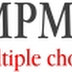1. The USART used as Serial Communication controller is
a.  8257
b.  8250
c.  8259
d.  8251

2. The UART used as Serial Communication controller is
a.  8257
b.  8250
c.  8259
d.  8251

3. The device from which data originates or terminates is called
a.  DTE
b.  DSR
c.  DCE
d.  DTR

4. The device which facilitates communications is called
a.  DTE
b.  DSR
c.  DCE
d.  DTR

5. Communications which take place in either direction between two systems, but can only occur in one direction at a time is known as
a. Full Duplex
b.  Simplex
c.  Half Duplex
d.  Complex

6. Each system can send and receive data at the same time is called
a.  Full Duplex
b.  Simplex
c.  Half Duplex
d.  Complex

7. Data line which can be transfer in only one direction is referred as as
a.  Full Duplex
b.  Simplex
c.  Half Duplex
d.  Complex

8. Synchronous serial data transmission sends data in which of these following ways ?
a.  First start bit, next 7 data bits, next parity bit followed
by 1 or 1 and a half stop bits
b.  A block of data bytes with start and end of blocks identifiedby a fixed pattern of bits
c.  A block of data bytes
d.  First 7 data bits followed by a parity bit

9. Asynchronous serial data transmission sends data in which of these following ways ?
a. First start bit, next 7 data bits, next parity bit followed by 1 or
1 and a half stop bits
b. A block of data bytes with start and end of blocks identified by a fixed pattern of bits c. A block of data bytes
d. First 7 data bits followed by a parity bit

10. If data bits are 7, parity is odd and 1 stop bit is transmitted how many bits are transmitted ?
a. 12
b.  11
c.  10
d.  9

11. Serial data is received by the DTE from the DCE through which one of these signals ? a. CTS
b.  CD
c.  RTS
d.  RxD

12. When the MODEM is ready to Transmit Data it Asserts which one of these signals is asserted ?
a. CTS
b.  CD
c.  RTS
d.  DSR

13. When the MODEM receives a signal that the remote modm is active it asserts which one of these signals ?
a. CTS
b.  CD
c.  RTS
d.  RxD

14. USART stands for which of the following ?
Transmitter
Transmitter
Transmitter

15. How many registers does 8251 have ?
a.  3
b.  2
c.  4
d.  1

16. The 8251 registers are accessed by which of the following signals ?
a. CLK
b.  TxC
c.  C/D
d.  SYNDET/BD

17. In the Synchronous mode the 8251 indicates that the specified Synchronous character is received ?
a. CLK
b.  TxC
c.  C/D
d.  SYNDET/BD

18. The clock used for Transmitting character is which one of the following ?
a. CLK
b.  TxC
c.  C/D
d.  SYNDET/BD

19. The 8251 gets its clock from which of these signals?
a.  CLK
b.  TxC
c.  C/D
d.  SYNDET/BD

20. When the DTE is ready to transmit data which one of these pins does it assert?
a. CTS
b.  CD
c.  RTS
d.  DSR

JNTU online bits MPMC ( Microprocessors and microcontrollers )Reviewed by Suresh Bojja on 10/23/2015 07:59:00 AM Rating: 5